$\mathrm{Ph}-\mathrm{CH}=\mathrm{CH}-\stackrel{\stackrel{\mathrm{O}}{||}}{\mathrm{C}}-{\mathrm{CH}}_{3}\to \mathrm{Ph}-\mathrm{CH}=\mathrm{CH}-{\mathrm{CO}}_{2}\mathrm{H}$
Above conversion can be achieved by :

(a)

(b)

(c) H2/Pt

(d) LiAlH4

Concept Questions :-

Preparation & properties of Aldehydes and Ketones
High Yielding Test Series + Question Bank - NEET 2020

Difficulty Level: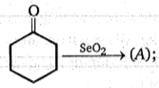Product (A) of the reaction is :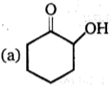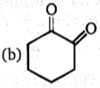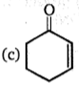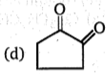Concept Questions :-

Preparation & properties of Aldehydes and Ketones
High Yielding Test Series + Question Bank - NEET 2020

Difficulty Level:

$R-\stackrel{\stackrel{\mathrm{O}}{\mathrm{ll}}}{\mathrm{C}}-\mathrm{H}\stackrel{\mathrm{R}-{\mathrm{NH}}_{2}}{\to }R-\mathrm{CH}=\mathrm{N}-R$. This reaction gives best yield at:

(a) pH 1 - 2

(b) pH 4 - 5

(c) pH 10 - 11

(d) pH 13 - 14

Concept Questions :-

Preparation & properties of Aldehydes and Ketones
High Yielding Test Series + Question Bank - NEET 2020

Difficulty Level:

An aromatic compound A of the molecular formula C8H10O on reaction with iodine and dilute NaOH gives a yellow precipitate. The structure of the compound is expected to be :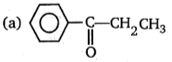(b) ${\mathrm{C}}_{6}{\mathrm{H}}_{5}{\mathrm{CHOHCH}}_{3}$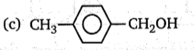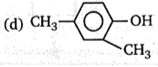Concept Questions :-

Preparation & properties of Aldehydes and Ketones
High Yielding Test Series + Question Bank - NEET 2020

Difficulty Level:

In the following sequence :

(a) ${\mathrm{CH}}_{3}{\mathrm{CH}}_{2}{\mathrm{CH}}_{2}{\mathrm{NH}}_{2}$

(b) ${\mathrm{CH}}_{3}{\mathrm{CH}}_{2}{\mathrm{CH}}_{2}{\mathrm{CONHCH}}_{3}$

(c) ${\mathrm{CH}}_{3}{\mathrm{CH}}_{2}{\mathrm{CH}}_{2}{\mathrm{NHCOCH}}_{3}$

(d) ${\mathrm{CH}}_{3}{\mathrm{CH}}_{2}{\mathrm{CH}}_{2}{\mathrm{CONHCOCH}}_{3}$

Concept Questions :-

Acid Derivatives, Preparation and Properties
High Yielding Test Series + Question Bank - NEET 2020

Difficulty Level:

Carbonyl compounds can generally be converted to hydrocarbons by :

(a) H2/Pt

(b) LiAlH4

(c) ${\mathrm{N}}_{2}{\mathrm{H}}_{4}-\mathrm{KOH}/∆$

(d) ${\mathrm{K}}_{2}{\mathrm{Cr}}_{2}{\mathrm{O}}_{7}-{\mathrm{H}}_{2}{\mathrm{SO}}_{4}$

Concept Questions :-

Preparation & properties of Aldehydes and Ketones
High Yielding Test Series + Question Bank - NEET 2020

Difficulty Level:

Which statement about the aldol condensation is correct ?

(a) A Lewis acid is commonly used as a catalyst

(b) The initial step is probably the formation of a carbanion

(c) A Lewis base is employed to induce carbocation formation

(d) The carbon chain is lengthened through the elimination of 1 mole of water

Concept Questions :-

Preparation & properties of Aldehydes and Ketones
High Yielding Test Series + Question Bank - NEET 2020

Difficulty Level:

A compound gives a positive test with I2/NaOH and is extracted from benzene by saturated NaHSO3, It may be :

(a) ${\mathrm{CH}}_{3}{\left({\mathrm{CH}}_{2}\right)}_{4}\mathrm{CHO}$

(b) ${\mathrm{CH}}_{3}{\left({\mathrm{CH}}_{2}\right)}_{3}{\mathrm{COCH}}_{3}$

(c) ${\mathrm{CH}}_{3}{\mathrm{CH}}_{2}{\mathrm{COCH}}_{2}{\mathrm{CH}}_{3}$

(d) ${\mathrm{CH}}_{3}{\left({\mathrm{CH}}_{2}\right)}_{4}{\mathrm{CH}}_{2}\mathrm{OH}$

Concept Questions :-

Preparation & properties of Aldehydes and Ketones
High Yielding Test Series + Question Bank - NEET 2020

Difficulty Level: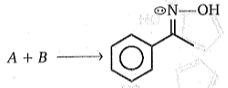Reactant (A) and (B) is:

(a)

(b)

(c)

(d)

Concept Questions :-

Preparation & properties of Aldehydes and Ketones
High Yielding Test Series + Question Bank - NEET 2020

Difficulty Level:

${\mathrm{CH}}_{3}-\stackrel{\stackrel{\mathrm{O}}{\mathrm{ll}}}{\mathrm{C}}-\mathrm{OH}\underset{∆}{\overset{\mathrm{Ca}{\left(\mathrm{OH}\right)}_{2}}{\to }}\left(\mathrm{A}\right)$

Product (A) is :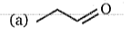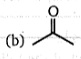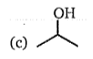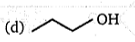Concept Questions :-

Carboxylic Acids